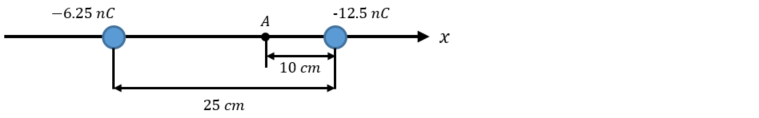Problem: Two point charges having charge of -6.25 x 10 -9 C and -12.5 x 10 -9 are 25.0 cm apart.a) Calculate the magnitude of Coulomb force between them. Is it repulsive or attractive?b) What is the magnitude of electric field at point A? What is its direction?

92% (37 ratings)
Problem Details

Two point charges having charge of -6.25 x 10 -9 C and -12.5 x 10 -9 are 25.0 cm apart.

a) Calculate the magnitude of Coulomb force between them. Is it repulsive or attractive?

b) What is the magnitude of electric field at point A? What is its direction?